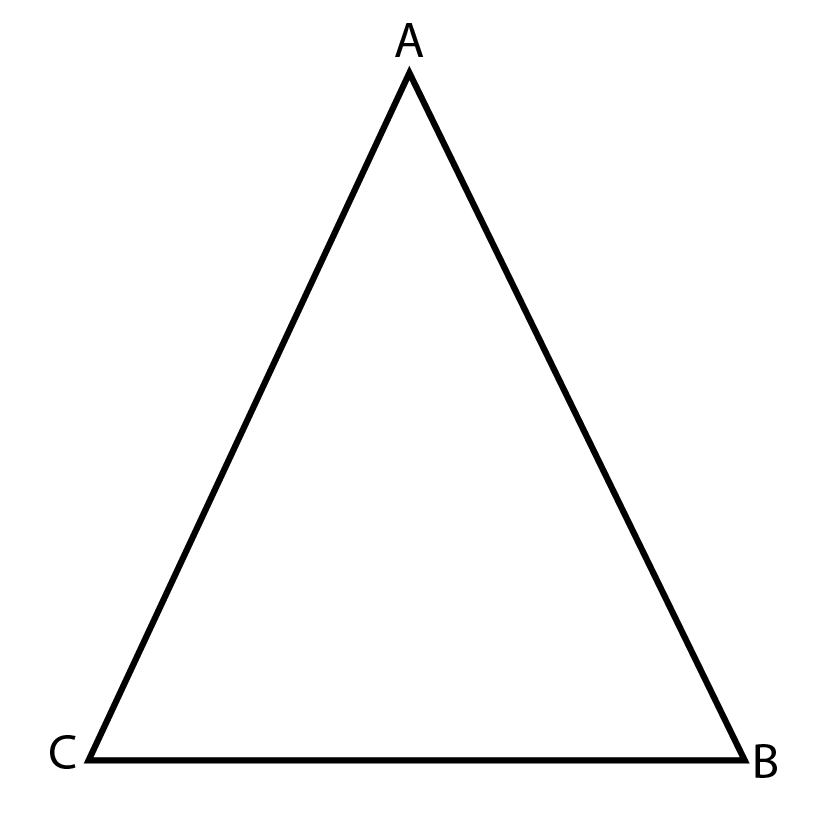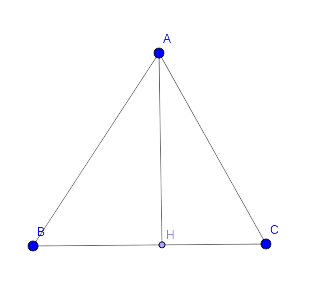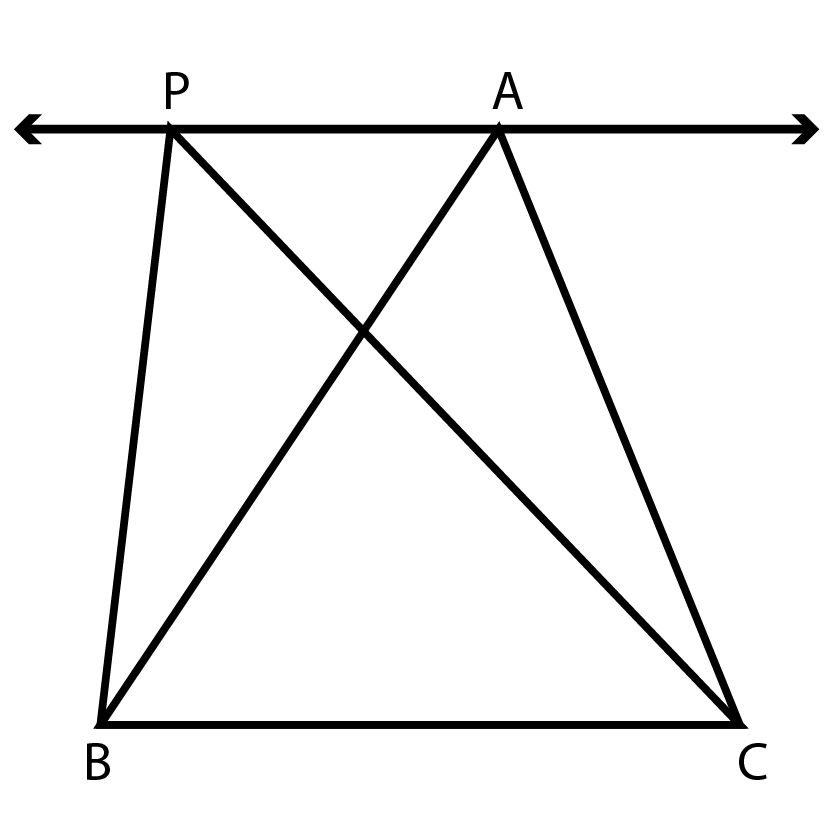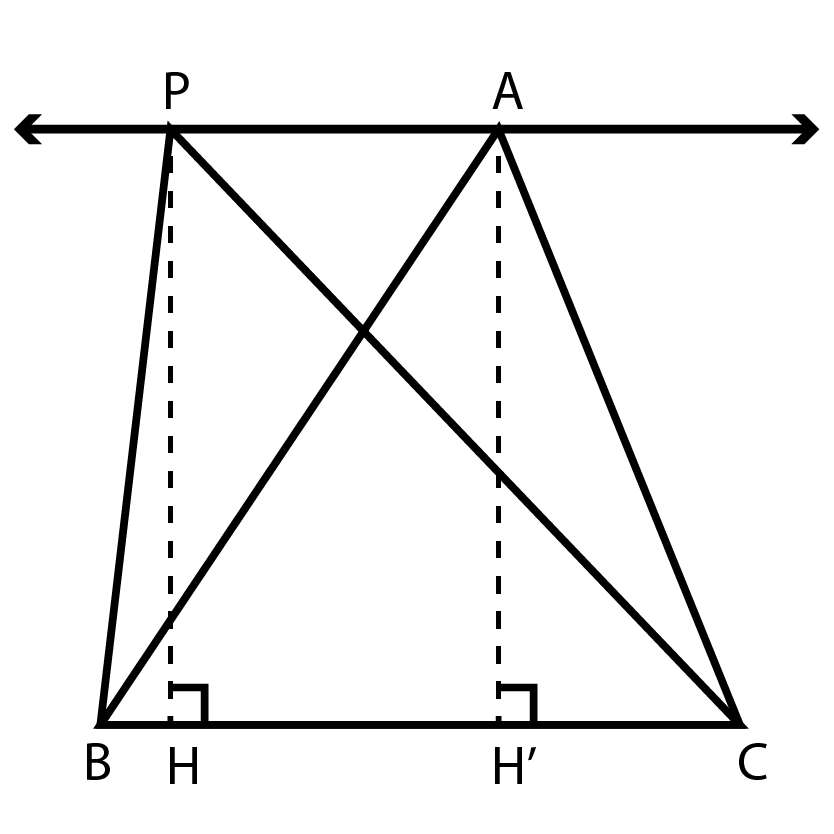Area of a triangles - same base and same parallels

## Objective

To verify that the triangles on the same base and between the same parallels are equal in area.

## Theory

### Triangle

A triangle is one of the basic shapes in geometry: a polygon with three corners or vertices and three sides or edges which are line segments. See Fig. (A).Fig. (A) A Triangle

### Area of trianglesFig. (B) A Triangle with height AH

Area (∆ABC) = ½ * Base * Perpendicular height

Here in the above figure, Fig. (B): A Triangle with height AH, Base = BC, and Perpendicular Height = AH

Therefore, Area (∆ABC) = ½ * BC * AH

### Parallel Lines

Parallel lines are lines in a plane that are always at the same distance apart. Parallel lines never intersect.

Distance between two parallel lines is always constant.

In Fig. (B), the line PA is parallel to the line BC. Here you can see how triangles can be on same base and between the same parallel lines.

BC is the common (or same) base for two triangles ABC and PBC in Fig. (B).

From the figure we can say that the base (BC) for both triangles is same/equal because both triangles are mounted on the same base.Fig. (C) Two triangles on the same base

From the Fig. (C), we can also say that the height of both triangles is same because triangles are in between two parallel lines and if two lines are parallel then distance between them is constant. Thus, the height of both triangles is same.Fig. (D) Two triangles on the same base and between the same parallels